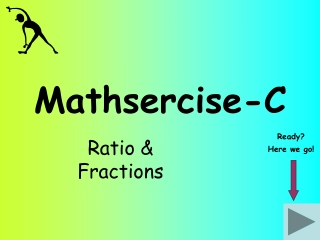DownloadDownload PresentationMathsercise-C

# Mathsercise-C

Télécharger la présentation## Mathsercise-C

- - - - - - - - - - - - - - - - - - - - - - - - - - - E N D - - - - - - - - - - - - - - - - - - - - - - - - - - -
##### Presentation Transcript

1. Mathsercise-C Ratio & Fractions Ready? Here we go!

2. Share £720 in the ratio 5:1 Question 2 5 + 1 = 6 720 ÷ 6 = £120 Add the parts of the ratios together to find out how many “parts” there are Check the total values add back to the original amount! ..then divide 720 into 6 parts 5 x £120 = £600 Answer 1 x £120 = £120 1 £600:£120 Ratio & Fractions

3. Share £320 in the ratio 1:3:4 Question 3 1 + 3 + 4 = 8 £320 ÷ 8 = £40 Check the total values add back to the original amount! Divide 320 into 8 parts Answer £40:£120:£160 2 Ratio & Fractions

4. As with adding fractions, you have to make the denominators the same 2 3 3 4 - 9 8 12 12 1 12 Work out… Question 4 x 3 x 4 Answer - 3 Ratio & Fractions

5. 5 8 2 9 x 10 5 72 36 Express … ..in its simplest form Start 5 x 2 = 10 Now simplify! 8 x 9 = 72 Answer = 4 Ratio & Fractions